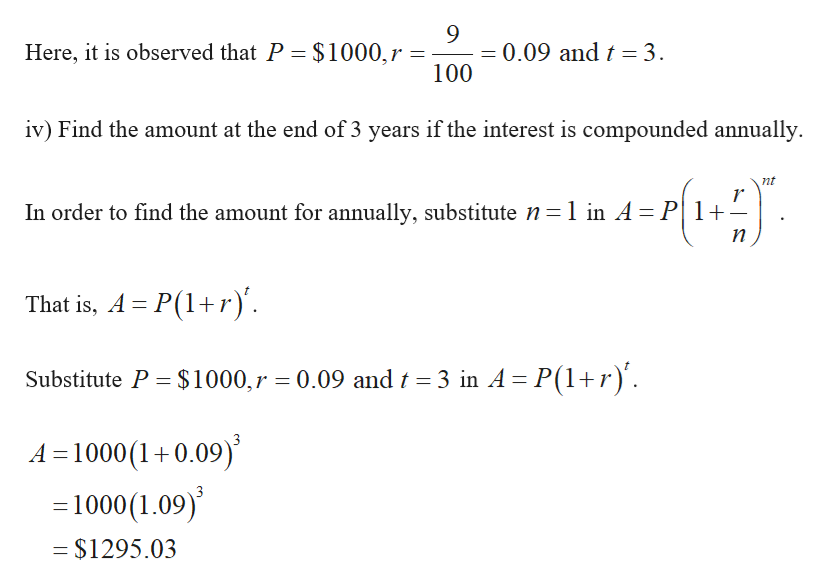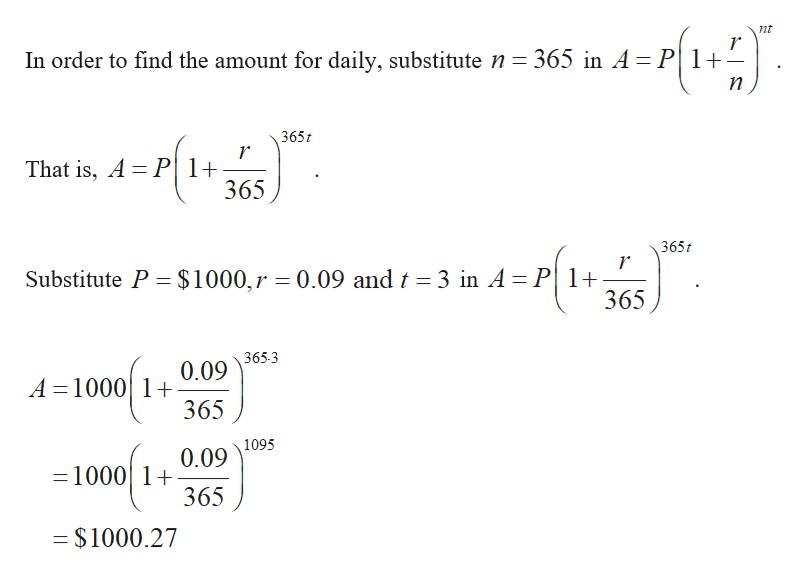# (a) If \$1000 is borrowed at 9% interest, find the amounts due at the end of 3 years if the interest is compounded as follows. (Round your answers to the nearest cent.)         (iv) weekly\$ (v) daily\$ (vi) hourly\$ (vii) continuously\$

Question
1 views

(a) If \$1000 is borrowed at 9% interest, find the amounts due at the end of 3 years if the interest is compounded as follows. (Round your answers to the nearest cent.)

 (iv) weekly \$ (v) daily \$ (vi) hourly \$ (vii) continuously \$
check_circle

Step 1

It is given that \$1000 is borrowed for 9% interest.

Step 2

Find the amounts due at the end of 3 years if the interest is compounded as follows.help_outlineImage Transcriptionclose= 0.09 and t = 3. 100 Here, it is observed that P = \$1000,r iv) Find the amount at the end of 3 years if the interest is compounded annually. nt In order to find the amount for annually, substitute n=1 in A= P|1+= п That is, A = P(1+r)'. Substitute P = \$1000,r = 0.09 and t = 3 in A = P(1+r)'. A =1000(1+0.09) = 1000(1.09) = \$1295.03 fullscreen
Step 3

v) Find the amount at the end of 3 years i...help_outlineImage Transcriptionclosent In order to find the amount for daily, substitute n= 365 in A=P|1+ п 365t That is, A = P|1+ 365 365t Substitute P = \$1000,r = 0.09 and t = 3 in A = P| 1+ 365 365-3 0.09 A = 1000| 1+ 365 1095 0.09 =1000| 1+ 365 = \$1000.27 fullscreen

### Want to see the full answer?

See Solution

#### Want to see this answer and more?

Solutions are written by subject experts who are available 24/7. Questions are typically answered within 1 hour.*

See Solution
*Response times may vary by subject and question.
Tagged in

### Other